Prealgebra

# 8.4Solve Equations with Fraction or Decimal Coefficients

Prealgebra8.4 Solve Equations with Fraction or Decimal Coefficients

### Learning Objectives

By the end of this section, you will be able to:
• Solve equations with fraction coefficients
• Solve equations with decimal coefficients

### Be Prepared 8.4

Before you get started, take this readiness quiz.

1. Multiply: $8·38.8·38.$
If you missed this problem, review Example 4.28
2. Find the LCD of $56and14.56and14.$
If you missed this problem, review Example 4.63
3. Multiply: $4.784.78$ by $100.100.$
If you missed this problem, review Example 5.18

### Solve Equations with Fraction Coefficients

Let’s use the General Strategy for Solving Linear Equations introduced earlier to solve the equation $18x+12=14.18x+12=14.$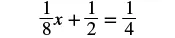To isolate the $xx$ term, subtract $1212$ from both sides.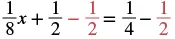Simplify the left side.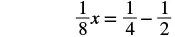Change the constants to equivalent fractions with the LCD.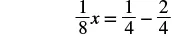Subtract.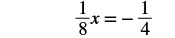Multiply both sides by the reciprocal of $1818$.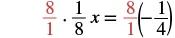Simplify.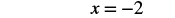This method worked fine, but many students don’t feel very confident when they see all those fractions. So we are going to show an alternate method to solve equations with fractions. This alternate method eliminates the fractions.

We will apply the Multiplication Property of Equality and multiply both sides of an equation by the least common denominator of all the fractions in the equation. The result of this operation will be a new equation, equivalent to the first, but with no fractions. This process is called clearing the equation of fractions. Let’s solve the same equation again, but this time use the method that clears the fractions.

### Example 8.37

Solve: $18x+12=14.18x+12=14.$

### Try It 8.73

Solve: $14x+12=58.14x+12=58.$

### Try It 8.74

Solve: $16y−13=16.16y−13=16.$

Notice in Example 8.37 that once we cleared the equation of fractions, the equation was like those we solved earlier in this chapter. We changed the problem to one we already knew how to solve! We then used the General Strategy for Solving Linear Equations.

### How To

#### Solve equations with fraction coefficients by clearing the fractions.

1. Step 1. Find the least common denominator of all the fractions in the equation.
2. Step 2. Multiply both sides of the equation by that LCD. This clears the fractions.
3. Step 3. Solve using the General Strategy for Solving Linear Equations.

### Example 8.38

Solve: $7=12x+34x−23x.7=12x+34x−23x.$

### Try It 8.75

Solve: $6=12v+25v−34v.6=12v+25v−34v.$

### Try It 8.76

Solve: $−1=12u+14u−23u.−1=12u+14u−23u.$

In the next example, we’ll have variables and fractions on both sides of the equation.

### Example 8.39

Solve: $x+13=16x−12.x+13=16x−12.$

### Try It 8.77

Solve: $a+34=38a−12.a+34=38a−12.$

### Try It 8.78

Solve: $c+34=12c−14.c+34=12c−14.$

In Example 8.40, we’ll start by using the Distributive Property. This step will clear the fractions right away!

### Example 8.40

Solve: $1=12(4x+2).1=12(4x+2).$

### Try It 8.79

Solve: $−11=12(6p+2).−11=12(6p+2).$

### Try It 8.80

Solve: $8=13(9q+6).8=13(9q+6).$

Many times, there will still be fractions, even after distributing.

### Example 8.41

Solve: $12(y−5)=14(y−1).12(y−5)=14(y−1).$

### Try It 8.81

Solve: $15(n+3)=14(n+2).15(n+3)=14(n+2).$

### Try It 8.82

Solve: $12(m−3)=14(m−7).12(m−3)=14(m−7).$

### Solve Equations with Decimal Coefficients

Some equations have decimals in them. This kind of equation will occur when we solve problems dealing with money and percent. But decimals are really another way to represent fractions. For example, $0.3=3100.3=310$ and $0.17=17100.0.17=17100.$ So, when we have an equation with decimals, we can use the same process we used to clear fractions—multiply both sides of the equation by the least common denominator.

### Example 8.42

Solve: $0.8x−5=7.0.8x−5=7.$

### Try It 8.83

Solve: $0.6x−1=11.0.6x−1=11.$

### Try It 8.84

Solve: $1.2x−3=9.1.2x−3=9.$

### Example 8.43

Solve: $0.06x+0.02=0.25x−1.5.0.06x+0.02=0.25x−1.5.$

### Try It 8.85

Solve: $0.14h+0.12=0.35h−2.4.0.14h+0.12=0.35h−2.4.$

### Try It 8.86

Solve: $0.65k−0.1=0.4k−0.35.0.65k−0.1=0.4k−0.35.$

The next example uses an equation that is typical of the ones we will see in the money applications in the next chapter. Notice that we will distribute the decimal first before we clear all decimals in the equation.

### Example 8.44

Solve: $0.25x+0.05(x+3)=2.85.0.25x+0.05(x+3)=2.85.$

### Try It 8.87

Solve: $0.25n+0.05(n+5)=2.95.0.25n+0.05(n+5)=2.95.$

### Try It 8.88

Solve: $0.10d+0.05(d−5)=2.15.0.10d+0.05(d−5)=2.15.$

### Section 8.4 Exercises

#### Practice Makes Perfect

Solve equations with fraction coefficients

In the following exercises, solve the equation by clearing the fractions.

209.

$1 4 x − 1 2 = − 3 4 1 4 x − 1 2 = − 3 4$

210.

$3 4 x − 1 2 = 1 4 3 4 x − 1 2 = 1 4$

211.

$5 6 y − 2 3 = − 3 2 5 6 y − 2 3 = − 3 2$

212.

$5 6 y − 1 3 = − 7 6 5 6 y − 1 3 = − 7 6$

213.

$1 2 a + 3 8 = 3 4 1 2 a + 3 8 = 3 4$

214.

$5 8 b + 1 2 = − 3 4 5 8 b + 1 2 = − 3 4$

215.

$2 = 1 3 x − 1 2 x + 2 3 x 2 = 1 3 x − 1 2 x + 2 3 x$

216.

$2 = 3 5 x − 1 3 x + 2 5 x 2 = 3 5 x − 1 3 x + 2 5 x$

217.

$1 4 m − 4 5 m + 1 2 m = −1 1 4 m − 4 5 m + 1 2 m = −1$

218.

$5 6 n − 1 4 n − 1 2 n = −2 5 6 n − 1 4 n − 1 2 n = −2$

219.

$x + 1 2 = 2 3 x − 1 2 x + 1 2 = 2 3 x − 1 2$

220.

$x + 3 4 = 1 2 x − 5 4 x + 3 4 = 1 2 x − 5 4$

221.

$1 3 w + 5 4 = w − 1 4 1 3 w + 5 4 = w − 1 4$

222.

$3 2 z + 1 3 = z − 2 3 3 2 z + 1 3 = z − 2 3$

223.

$1 2 x − 1 4 = 1 12 x + 1 6 1 2 x − 1 4 = 1 12 x + 1 6$

224.

$1 2 a − 1 4 = 1 6 a + 1 12 1 2 a − 1 4 = 1 6 a + 1 12$

225.

$1 3 b + 1 5 = 2 5 b − 3 5 1 3 b + 1 5 = 2 5 b − 3 5$

226.

$1 3 x + 2 5 = 1 5 x − 2 5 1 3 x + 2 5 = 1 5 x − 2 5$

227.

$1 = 1 6 ( 12 x − 6 ) 1 = 1 6 ( 12 x − 6 )$

228.

$1 = 1 5 ( 15 x − 10 ) 1 = 1 5 ( 15 x − 10 )$

229.

$1 4 ( p − 7 ) = 1 3 ( p + 5 ) 1 4 ( p − 7 ) = 1 3 ( p + 5 )$

230.

$1 5 ( q + 3 ) = 1 2 ( q − 3 ) 1 5 ( q + 3 ) = 1 2 ( q − 3 )$

231.

$1 2 ( x + 4 ) = 3 4 1 2 ( x + 4 ) = 3 4$

232.

$1 3 ( x + 5 ) = 5 6 1 3 ( x + 5 ) = 5 6$

Solve Equations with Decimal Coefficients

In the following exercises, solve the equation by clearing the decimals.

233.

$0.6 y + 3 = 9 0.6 y + 3 = 9$

234.

$0.4 y − 4 = 2 0.4 y − 4 = 2$

235.

$3.6 j − 2 = 5.2 3.6 j − 2 = 5.2$

236.

$2.1 k + 3 = 7.2 2.1 k + 3 = 7.2$

237.

$0.4 x + 0.6 = 0.5 x − 1.2 0.4 x + 0.6 = 0.5 x − 1.2$

238.

$0.7 x + 0.4 = 0.6 x + 2.4 0.7 x + 0.4 = 0.6 x + 2.4$

239.

$0.23 x + 1.47 = 0.37 x − 1.05 0.23 x + 1.47 = 0.37 x − 1.05$

240.

$0.48 x + 1.56 = 0.58 x − 0.64 0.48 x + 1.56 = 0.58 x − 0.64$

241.

$0.9 x − 1.25 = 0.75 x + 1.75 0.9 x − 1.25 = 0.75 x + 1.75$

242.

$1.2 x − 0.91 = 0.8 x + 2.29 1.2 x − 0.91 = 0.8 x + 2.29$

243.

$0.05 n + 0.10 ( n + 8 ) = 2.15 0.05 n + 0.10 ( n + 8 ) = 2.15$

244.

$0.05 n + 0.10 ( n + 7 ) = 3.55 0.05 n + 0.10 ( n + 7 ) = 3.55$

245.

$0.10 d + 0.25 ( d + 5 ) = 4.05 0.10 d + 0.25 ( d + 5 ) = 4.05$

246.

$0.10 d + 0.25 ( d + 7 ) = 5.25 0.10 d + 0.25 ( d + 7 ) = 5.25$

247.

$0.05 ( q − 5 ) + 0.25 q = 3.05 0.05 ( q − 5 ) + 0.25 q = 3.05$

248.

$0.05 ( q − 8 ) + 0.25 q = 4.10 0.05 ( q − 8 ) + 0.25 q = 4.10$

#### Everyday Math

249.

Coins Taylor has $2.002.00$ in dimes and pennies. The number of pennies is $22$ more than the number of dimes. Solve the equation $0.10d+0.01(d+2)=20.10d+0.01(d+2)=2$ for $d,d,$ the number of dimes.

250.

Stamps Travis bought $9.459.45$ worth of $49-cent49-cent$ stamps and $21-cent21-cent$ stamps. The number of $21-cent21-cent$ stamps was $55$ less than the number of $49-cent49-cent$ stamps. Solve the equation $0.49s+0.21(s−5)=9.450.49s+0.21(s−5)=9.45$ for $s,s,$ to find the number of $49-cent49-cent$ stamps Travis bought.

#### Writing Exercises

251.

Explain how to find the least common denominator of $38,16,and23.38,16,and23.$

252.

If an equation has several fractions, how does multiplying both sides by the LCD make it easier to solve?

253.

If an equation has fractions only on one side, why do you have to multiply both sides of the equation by the LCD?

254.

In the equation $0.35x+2.1=3.85,0.35x+2.1=3.85,$ what is the LCD? How do you know?

#### Self Check

After completing the exercises, use this checklist to evaluate your mastery of the objectives of this section.

Overall, after looking at the checklist, do you think you are well-prepared for the next Chapter? Why or why not?

Order a print copy

As an Amazon Associate we earn from qualifying purchases.